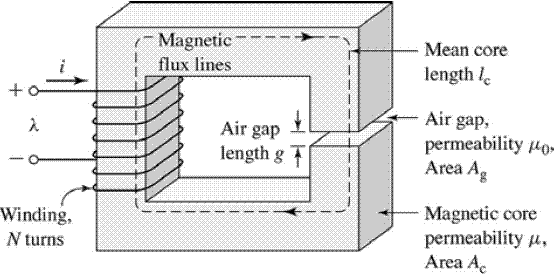# Why Do We Need an Air Gap in Magnetic Circuit & How to Calculate it?

In this blog we will review the theory of the Air gaps in magnetic circuits. A magnetic circuit is where a magnetic flux is circulated or follow through a closed area or path. An air gap is a non-magnetic part of a magnetic circuits and it is usually connected magnetically in series with the rest of the circuit. This allows a substantial part of the magnetic flux flows through the gap. Depending on application, air gap may be filled with a non-magnetic material such as gas, water, vacuum, plastic, wood etc. and not necessarily just with air. Well, what exactly is the function of the air gap?

## Why Do We Need Air Gap in a Magnetic Circuit?

Consider a magnetic circuit with an air gap as below. Let’s discuss different reasons why air gaps are crucial in practical applications. In this circuit, there is only one path for the magnetic circuit and thus it can be called as a series magnetic circuit.Fig 1. Series Magnetic Circuit with an Air gap

One of main reasons for an Air Gap is to increase the reluctance of the magnetic circuit. The amount of air or another non-magnetic material like a fibre plate or fibre board increases the reluctance of the circuit, thereby increasing the amount of current that we could put in a coil before we reach saturation. Also, the air gaps help the magnetic flux to expand outside the magnetic circuit. This flux fringes out into the neighbouring air path and such paths for flux are called flux fringing resulting in a non-uniform flux density in the air gap. As we increase the air gap, it increases flux fringing and vice versa. With small air gaps, fringing can be neglected unless otherwise noted. However, with a larger air gap we might have to take that change in area into consideration when we start doing flux density calculations.

## How to Calculate Flux Density in an Air Gap?

Let’s look at the basic formula to calculate flux density.

Flux density, Bg = Φg / Ag

where, B is magnetic flux density in Teslas (T)

Φ is magnetic flux in Webers (Wb)

A is area in square meters (m2)

As we know that permeability of any material can be stated as the ratio of the magnetic flux density to the magnetic field intensity of a material. It can be expressed as below,

μ = B / H

where, μ is permeability of a material, in henrys/meter

H is magnetic field strength, in ampere-turns/meter

B is flux density, in teslas

Solving the above equation for B, we get

B = μ x H                        ….. (Equation 1)

Now, magnetizing force of the air gap is determined as below,

H = Fm / l                        ….. (Equation 2)

where, Fm is Magnetomotive force (mmf), in ampere-turns, l is length of material (gap), in meters

Substituting the value of H from Equation 2  into Equation 1, we get

B = μ x (Fm/l)

Solving the above equation for magnetomotive force, we get

Fm = Bl / μ                  ........ (Equation 3)

Let’s assume that we have an air gap, and the permeability of air (free space) is a constant

μair = 4π x 10-7  H/m

Replacing the value for μ in Equation 3, we come up with a very basic formula for determining the magnetomotive force required to set up a particular flux in an air gap.

Fm = BL / (4π x 10-7)

The air gap can take different form, shape and size depending on the type of magnetic circuit and its shape. In some circuits it might be actually an integral part ensuring correct performance of the device, but in other cases it should be as small as possible. Such requirements will be determined by the operating principle, performance, size, efficiency, and many other technological factors.

We hope this has been helpful to you as a Technician or a student entering the field. If you have any questions about the Electronics or the Electromechanical Technician programs you can reach one of our Program Consultants toll-free at 1-888-553-5333 or by email at info@gbctechtraining.com.

### Air gap in ferite transformer

Submitted by Sigitas (not verified) on Sun, 09/26/2021 - 11:14

How to determine if a transformer core is with an air gap or without air gap?

### Data sheets on the…

Submitted by Iris on Mon, 09/27/2021 - 15:58

Data sheets on the transformer you are considering would be the source for all parameters relating to the xformer. With air gap xformers it can be visible. Product data sheets from the vendor is the answer.

### Thank you for that good…

Submitted by Anonymous (not verified) on Fri, 12/31/2021 - 02:51

Thank you for that good explanation